• Slides: 23COMP 171 Fall 2006 Linked Lists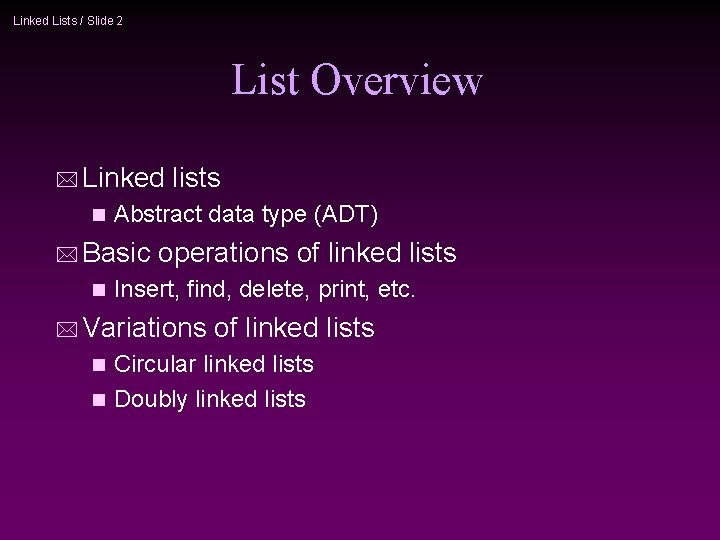Linked Lists / Slide 2 List Overview * Linked n Abstract data type (ADT) * Basic n lists operations of linked lists Insert, find, delete, print, etc. * Variations of linked lists Circular linked lists n Doubly linked lists nLinked Lists / Slide 3 Linked Lists A B C Head *A linked list is a series of connected nodes * Each node contains at least A piece of data (any type) n Pointer to the next node in the list n * Head: pointer to the first node * The last node points to NULL node A data pointer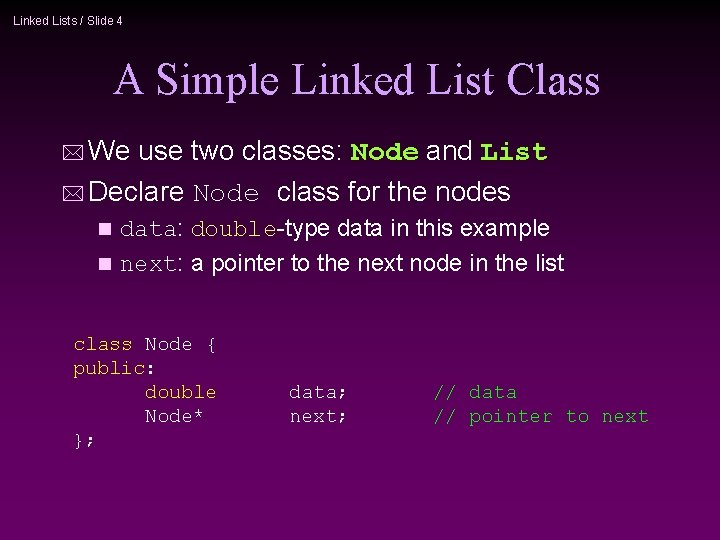Linked Lists / Slide 4 A Simple Linked List Class * We use two classes: Node and List * Declare Node class for the nodes data: double-type data in this example n next: a pointer to the next node in the list n class Node { public: double Node* }; data; next; // data // pointer to next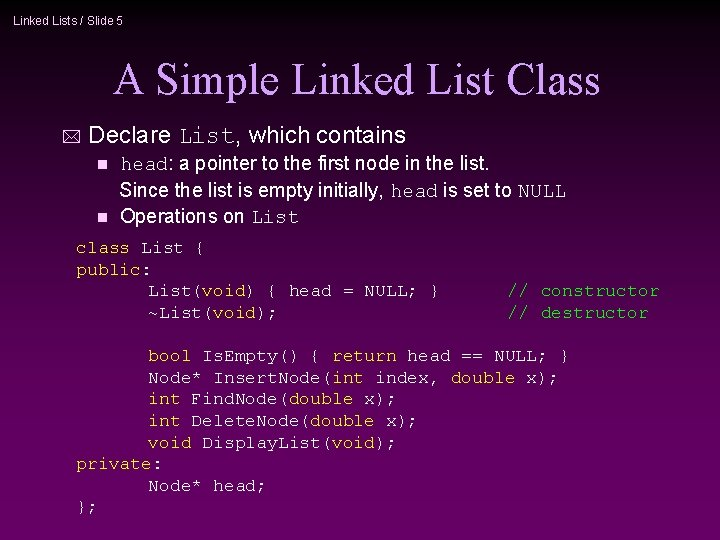Linked Lists / Slide 5 A Simple Linked List Class * Declare List, which contains head: a pointer to the first node in the list. Since the list is empty initially, head is set to NULL n Operations on List n class List { public: List(void) { head = NULL; } ~List(void); // constructor // destructor bool Is. Empty() { return head == NULL; } Node* Insert. Node(int index, double x); int Find. Node(double x); int Delete. Node(double x); void Display. List(void); private: Node* head; };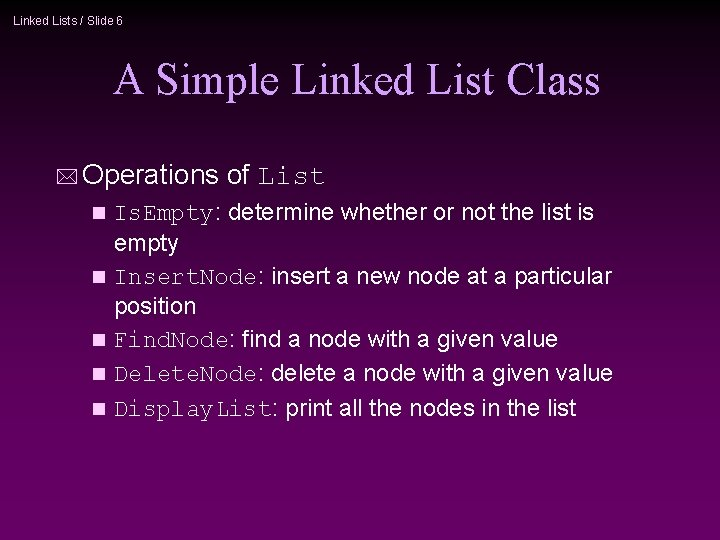Linked Lists / Slide 6 A Simple Linked List Class * Operations n n n of List Is. Empty: determine whether or not the list is empty Insert. Node: insert a new node at a particular position Find. Node: find a node with a given value Delete. Node: delete a node with a given value Display. List: print all the nodes in the list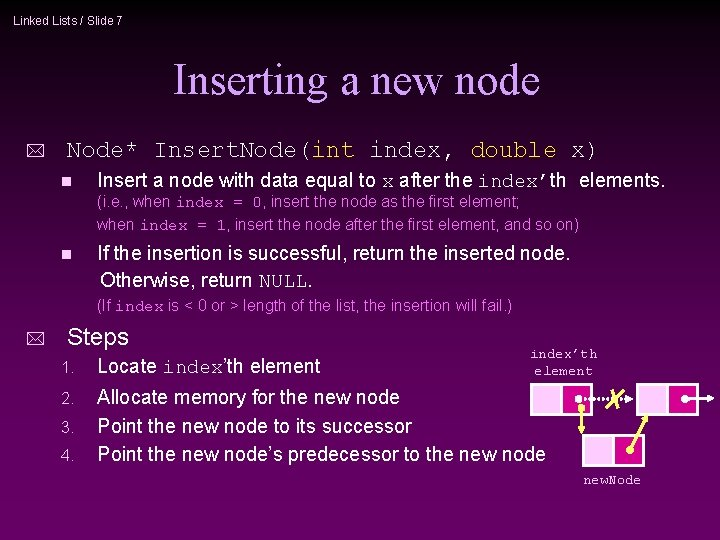Linked Lists / Slide 7 Inserting a new node * Node* Insert. Node(int index, double x) n Insert a node with data equal to x after the index’th elements. (i. e. , when index = 0, insert the node as the first element; when index = 1, insert the node after the first element, and so on) n If the insertion is successful, return the inserted node. Otherwise, return NULL. (If index is < 0 or > length of the list, the insertion will fail. ) * Steps index’th element 1. Locate index’th element 2. Allocate memory for the new node Point the new node to its successor Point the new node’s predecessor to the new node 3. 4. new. Node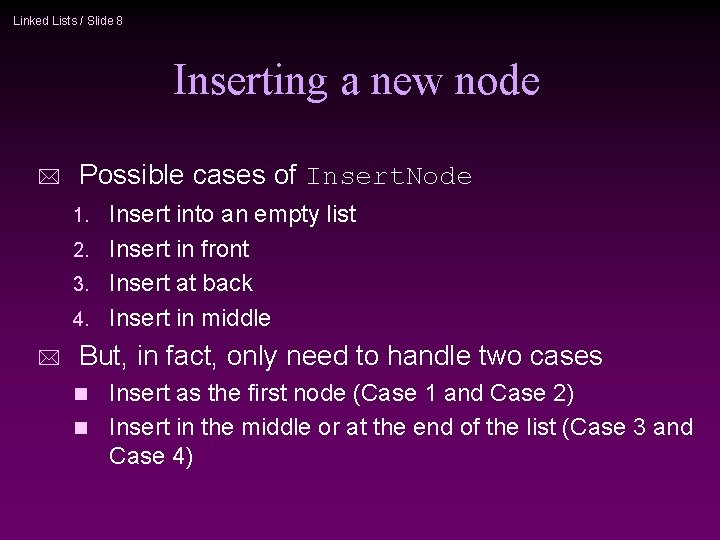Linked Lists / Slide 8 Inserting a new node * Possible cases of Insert. Node Insert into an empty list 2. Insert in front 3. Insert at back 4. Insert in middle 1. * But, in fact, only need to handle two cases Insert as the first node (Case 1 and Case 2) n Insert in the middle or at the end of the list (Case 3 and Case 4) nLinked Lists / Slide 9 Inserting a new node Node* List: : Insert. Node(int index, double x) { if (index < 0) return NULL; Try to locate index’th node. If it doesn’t exist, return NULL. int curr. Index = 1; Node* curr. Node = head; while (curr. Node && index > curr. Index) { curr. Node = curr. Node->next; curr. Index++; } if (index > 0 && curr. Node == NULL) return NULL; Node* new. Node = new. Node->data = if (index == 0) { new. Node->next head } else { new. Node->next curr. Node->next } return new. Node; } new x; Node; = = head; new. Node; = = curr. Node->next; new. Node;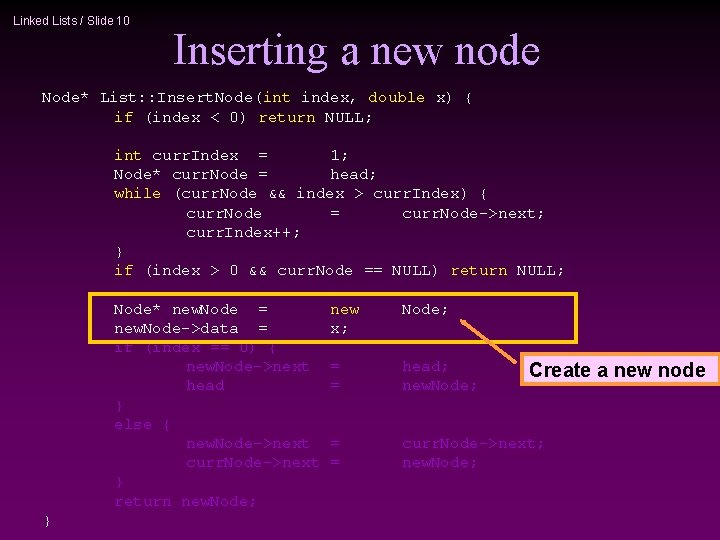Linked Lists / Slide 10 Inserting a new node Node* List: : Insert. Node(int index, double x) { if (index < 0) return NULL; int curr. Index = 1; Node* curr. Node = head; while (curr. Node && index > curr. Index) { curr. Node = curr. Node->next; curr. Index++; } if (index > 0 && curr. Node == NULL) return NULL; Node* new. Node = new. Node->data = if (index == 0) { new. Node->next head } else { new. Node->next curr. Node->next } return new. Node; } new x; Node; = = head; new. Node; = = curr. Node->next; new. Node; Create a new nodeLinked Lists / Slide 11 Inserting a new node Node* List: : Insert. Node(int index, double x) { if (index < 0) return NULL; int curr. Index = 1; Node* curr. Node = head; while (curr. Node && index > curr. Index) { curr. Node = curr. Node->next; curr. Index++; } if (index > 0 && curr. Node == NULL) return NULL; Node* new. Node = new. Node->data = if (index == 0) { new. Node->next head } else { new. Node->next curr. Node->next } return new. Node; } new x; Node; = = head; new. Node; = = curr. Node->next; new. Node; Insert as first element head new. Node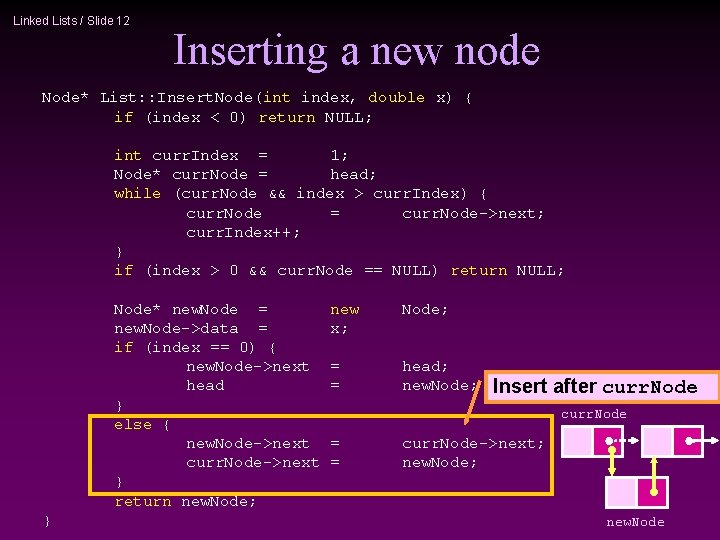Linked Lists / Slide 12 Inserting a new node Node* List: : Insert. Node(int index, double x) { if (index < 0) return NULL; int curr. Index = 1; Node* curr. Node = head; while (curr. Node && index > curr. Index) { curr. Node = curr. Node->next; curr. Index++; } if (index > 0 && curr. Node == NULL) return NULL; Node* new. Node = new. Node->data = if (index == 0) { new. Node->next head } else { new. Node->next curr. Node->next } return new. Node; } new x; Node; = = head; new. Node; Insert after curr. Node = = curr. Node->next; new. Node; new. Node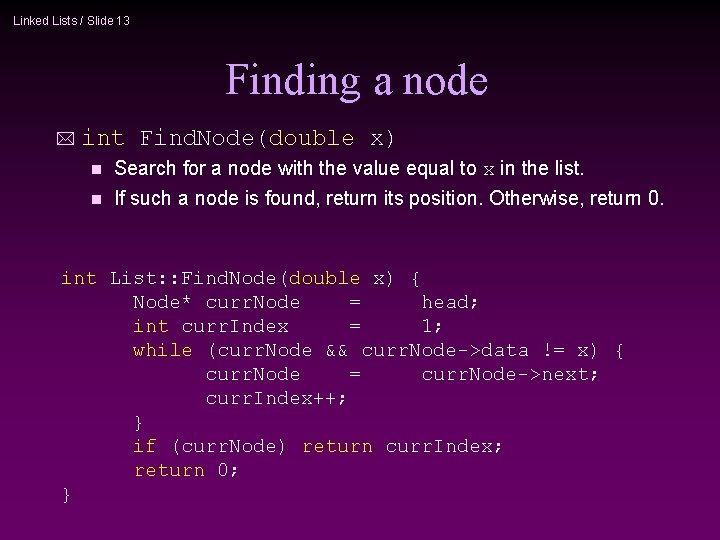Linked Lists / Slide 13 Finding a node * int Find. Node(double x) n Search for a node with the value equal to x in the list. n If such a node is found, return its position. Otherwise, return 0. int List: : Find. Node(double x) { Node* curr. Node = head; int curr. Index = 1; while (curr. Node && curr. Node->data != x) { curr. Node = curr. Node->next; curr. Index++; } if (curr. Node) return curr. Index; return 0; }Linked Lists / Slide 14 * int Delete. Node(double x) n Delete a node with the value equal to x from the list. n * Deleting a node If such a node is found, return its position. Otherwise, return 0. Steps n Find the desirable node (similar to Find. Node) Release the memory occupied by the found node n Set the pointer of the predecessor of the found node to the successor of the found node n * Like Insert. Node, there are two special cases Delete first node n Delete the node in middle or at the end of the list nLinked Lists / Slide 15 Deleting a node int List: : Delete. Node(double x) { Try to find the node Node* prev. Node = NULL; its value equal to x Node* curr. Node = head; int curr. Index = 1; while (curr. Node && curr. Node->data != x) { prev. Node = curr. Node; curr. Node = curr. Node->next; curr. Index++; } if (curr. Node) { if (prev. Node) { prev. Node->next = curr. Node->next; delete curr. Node; } else { head = curr. Node->next; delete curr. Node; } return curr. Index; } return 0; } with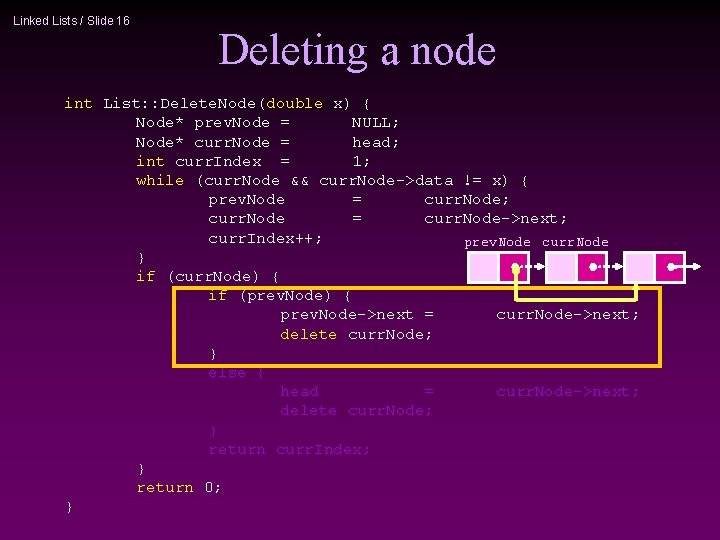Linked Lists / Slide 16 Deleting a node int List: : Delete. Node(double x) { Node* prev. Node = NULL; Node* curr. Node = head; int curr. Index = 1; while (curr. Node && curr. Node->data != x) { prev. Node = curr. Node; curr. Node = curr. Node->next; curr. Index++; prev. Node curr. Node } if (curr. Node) { if (prev. Node) { prev. Node->next = curr. Node->next; delete curr. Node; } else { head = curr. Node->next; delete curr. Node; } return curr. Index; } return 0; }Linked Lists / Slide 17 Deleting a node int List: : Delete. Node(double x) { Node* prev. Node = NULL; Node* curr. Node = head; int curr. Index = 1; while (curr. Node && curr. Node->data != x) { prev. Node = curr. Node; curr. Node = curr. Node->next; curr. Index++; } if (curr. Node) { if (prev. Node) { prev. Node->next = curr. Node->next; delete curr. Node; } else { head = curr. Node->next; delete curr. Node; } return curr. Index; } head curr. Node return 0; }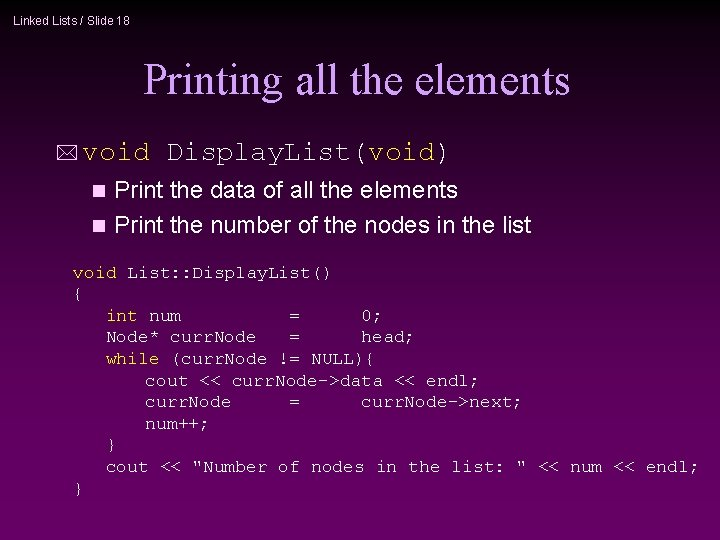Linked Lists / Slide 18 Printing all the elements * void Display. List(void) Print the data of all the elements n Print the number of the nodes in the list n void List: : Display. List() { int num = 0; Node* curr. Node = head; while (curr. Node != NULL){ cout << curr. Node->data << endl; curr. Node = curr. Node->next; num++; } cout << "Number of nodes in the list: " << num << endl; }Linked Lists / Slide 19 Destroying the list * ~List(void) Use the destructor to release all the memory used by the list. n Step through the list and delete each node one by one. n List: : ~List(void) { Node* curr. Node = head, *next. Node = NULL; while (curr. Node != NULL) { next. Node = curr. Node->next; // destroy the current node delete curr. Node; curr. Node = next. Node; } }Linked Lists / Slide 20 6 7 5 Number of nodes in the list: 3 5. 0 found 4. 5 not found 6 5 Number of nodes in the list: 2 result Using List int main(void) { List list; list. Insert. Node(0, 7. 0); list. Insert. Node(1, 5. 0); list. Insert. Node(-1, 5. 0); list. Insert. Node(0, 6. 0); list. Insert. Node(8, 4. 0); // print all the elements list. Display. List(); if(list. Find. Node(5. 0) > 0) else if(list. Find. Node(4. 5) > 0) else list. Delete. Node(7. 0); list. Display. List(); return 0; } // // // successful unsuccessful cout << << "5. 0 "4. 5 found" << endl; not found" << endl;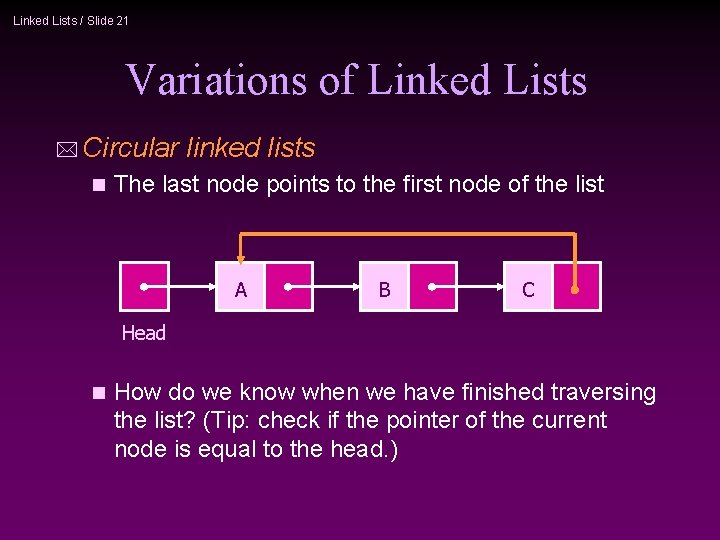Linked Lists / Slide 21 Variations of Linked Lists * Circular n linked lists The last node points to the first node of the list A B C Head n How do we know when we have finished traversing the list? (Tip: check if the pointer of the current node is equal to the head. )Linked Lists / Slide 22 Variations of Linked Lists * Doubly linked lists Each node points to not only successor but the predecessor n There are two NULL: at the first and last nodes in the list n Advantage: given a node, it is easy to visit its predecessor. Convenient to traverse lists backwards n A Head B CLinked Lists / Slide 23 Array versus Linked Lists * Linked lists are more complex to code and manage than arrays, but they have some distinct advantages. n Dynamic: a linked list can easily grow and shrink in size. 1 We don’t need to know how many nodes will be in the list. They are created in memory as needed. 1 In contrast, the size of a C++ array is fixed at compilation time. n Easy and fast insertions and deletions 1 To insert or delete an element in an array, we need to copy to temporary variables to make room for new elements or close the gap caused by deleted elements. 1 With a linked list, no need to move other nodes. Only need to reset some pointers.